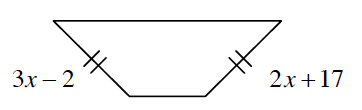### Home > GC > Chapter 1 > Lesson 1.3.2 > Problem1-114

1-114.

Multiple Choice: Which equation below correctly represents the relationship of the sides given in the diagram at right?1. $3x-2+2x+17=360º$

1. $3x-2+2x+17=180º$

1. $3x-2+2x+17=90º$

1. $3x-2=2x+17$

Remember that sides with the same marks have the same length.

(d)